Updating search results...

# 44 Results

View
Selected filters:
• Calculus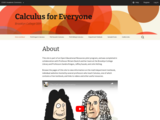Conditional Remix & Share Permitted
CC BY-NC-SA
Rating
0.0 stars

Browse the pages of this site to view information on the math department textbook, individual websites hosted by several professors who teach Calculus, one of which contains a free textbook, and links to videos and other useful resources.

This site is part of an Open Educational Resources pilot program, and was completed in collaboration with Professor Miriam Deutch and her team at the Brooklyn College Library and Professors Sandra Kingan, Jeffrey Suzuki, and John Velling.

Subject:
Calculus
Mathematics
Material Type:
Full Course
Homework/Assignment
Textbook
Provider:
CUNY
Provider Set:
Brooklyn College
Author:
Jeff Suzuki
John Velling
Miriam Deutch
Sandra Kingan
11/15/2021Only Sharing Permitted
CC BY-NC-ND
Rating
0.0 stars

Professor Kingan’s motivation for writing her free Calculus I textbook was to help address the departments high failure rates in Calculus. Along with another CUNY initiative to offer Calculus workshops in advance of taking the course, Kingan’s concise textbook in Calculus I offers students inside and outside of CUNY an opportunity to prepare for Calculus I at their own pace. She also believes that by providing free access to this material she could help to overcome some of the inequity students experience when Calculus is not offered in their high school. The textbook was written specifically for this pilot project.
The full website is available at https://calculusforeveryoneoer.commons.gc.cuny.edu/ and was developed in collaboration with Miriam Deutch and Diane Dias de Fazio.

Subject:
Calculus
Mathematics
Material Type:
Textbook
Provider:
Provider Set:
Brooklyn College
Author:
Brooklyn College Library and Academic IT
Kingan, Sandra
01/01/2015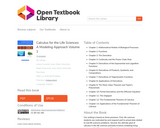Conditional Remix & Share Permitted
CC BY-NC-SA
Rating
0.0 stars

Our writing is based on three premises. First, life sciences students are motivated by and respond well to actual data related to real life sciences problems. Second, the ultimate goal of calculus in the life sciences primarily involves modeling living systems with difference and differential equations. Understanding the concepts of derivative and integral are crucial, but the ability to compute a large array of derivatives and integrals is of secondary importance. Third, the depth of calculus for life sciences students should be comparable to that of the traditional physics and engineering calculus course; else life sciences students will be short changed and their faculty will advise them to take the 'best' (engineering) course.

Subject:
Calculus
Mathematics
Material Type:
Textbook
Provider:
University of Minnesota
Provider Set:
Open Textbook Library
Author:
James L. Cornette
Ralph A. Ackerman
01/01/2012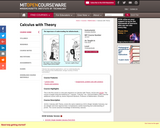Conditional Remix & Share Permitted
CC BY-NC-SA
Rating
0.0 stars

Calculus with Theory, covers the same material as 18.01 (Single Variable Calculus), but at a deeper and more rigorous level. It emphasizes careful reasoning and understanding of proofs. The course assumes knowledge of elementary calculus.

Subject:
Calculus
Mathematics
Material Type:
Full Course
Provider:
M.I.T.
Provider Set:
M.I.T. OpenCourseWare
Author:
Breiner, Christine
01/01/2010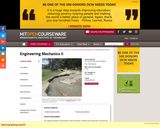Conditional Remix & Share Permitted
CC BY-NC-SA
Rating
0.0 stars

This subject provides an introduction to fluid mechanics. Students are introduced to and become familiar with all relevant physical properties and fundamental laws governing the behavior of fluids and learn how to solve a variety of problems of interest to civil and environmental engineers. While there is a chance to put skills from Calculus and Differential Equations to use in this subject, the emphasis is on physical understanding of why a fluid behaves the way it does. The aim is to make the students think as a fluid. In addition to relating a working knowledge of fluid mechanics, the subject prepares students for higher-level subjects in fluid dynamics.

Subject:
Applied Science
Calculus
Environmental Science
Mathematics
Material Type:
Full Course
Provider:
M.I.T.
Provider Set:
M.I.T. OpenCourseWare
Author:
01/01/2006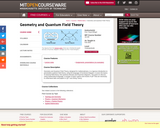Conditional Remix & Share Permitted
CC BY-NC-SA
Rating
0.0 stars

A rigorous introduction designed for mathematicians into perturbative quantum field theory, using the language of functional integrals. Basics of classical field theory. Free quantum theories. Feynman diagrams. Renormalization theory. Local operators. Operator product expansion. Renormalization group equation. The goal is to discuss, using mathematical language, a number of basic notions and results of QFT that are necessary to understand talks and papers in QFT and string theory.

Subject:
Calculus
Geometry
Mathematics
Material Type:
Full Course
Provider:
M.I.T.
Provider Set:
M.I.T. OpenCourseWare
Author:
Etingof, Pavel I.
01/01/2002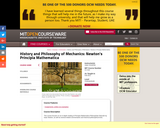Conditional Remix & Share Permitted
CC BY-NC-SA
Rating
0.0 stars

This course focuses on an in-depth reading of Principia Mathematica Philosophiae Naturalis by Isaac Newton, as well as several related commentaries and historical philosophical texts.

Subject:
Arts and Humanities
Calculus
Mathematics
Philosophy
Material Type:
Full Course
Provider:
M.I.T.
Provider Set:
M.I.T. OpenCourseWare
Author:
01/01/2011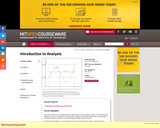Conditional Remix & Share Permitted
CC BY-NC-SA
Rating
0.0 stars

Analysis I in its various versions covers fundamentals of mathematical analysis: continuity, differentiability, some form of the Riemann integral, sequences and series of numbers and functions, uniform convergence with applications to interchange of limit operations, some point-set topology, including some work in Euclidean n-space. MIT students may choose to take one of three versions of 18.100: Option A (18.100A) chooses less abstract definitions and proofs, and gives applications where possible. Option B (18.100B) is more demanding and for students with more mathematical maturity; it places more emphasis from the beginning on point-set topology and n-space, whereas Option A is concerned primarily with analysis on the real line, saving for the last weeks work in 2-space (the plane) and its point-set topology. Option C (18.100C) is a 15-unit variant of Option B, with further instruction and practice in written and oral communication.

Subject:
Calculus
Mathematics
Material Type:
Full Course
Provider:
M.I.T.
Provider Set:
M.I.T. OpenCourseWare
Author:
Arthur Mattuck
01/01/2012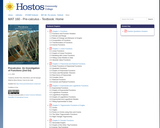Conditional Remix & Share Permitted
CC BY-SA
Rating
0.0 stars

Precalculus: An Ivestigation of Functions. Chapters cover: Functions, Linear Functions, Polynormal and Rational Functions, Exponential and Logarithmic Functions, Trigonometric Functions of Angles, Periodic Functions, Trigonometric Equations and Identities, Further Applications of Trigonometry, and Conics.

Subject:
Calculus
Mathematics
Material Type:
Textbook
Provider:
CUNY
Provider Set:
Hostos Community College
Author:
David Lippman
Melonie Rasmussen
04/03/2020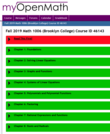Conditional Remix & Share Permitted
CC BY-NC-SA
Rating
0.0 stars

Real Numbers, Sets and Intervals, Absolute Value, Exponents and Radicals, Algebraic Expressions, Polynomials, Rational Expressions, Factoring, Solving Basic Equations, Solving Equations Involving Radicals, Solving Quadratic Equations, The Coordinate Plane, Lines, Introduction to Functions and Relations, Linear Functions in Two Variables, Systems of Linear Equations, Graphs of linear and quadratic functions. Starting Fall 2019 qualifies as STEM variant course - Satisfies Pathways Required Core Math and Quantitative Reasoning requirement.

Subject:
Algebra
Calculus
Mathematics
Material Type:
Activity/Lab
Homework/Assignment
Interactive
Lesson
Provider:
CUNY
Provider Set:
Brooklyn College
Author:
Amy Wolfe
Jeff Suzuki
03/08/2021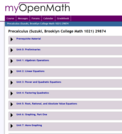Conditional Remix & Share Permitted
CC BY-NC-SA
Rating
0.0 stars

Preparation for calculus with more introductory material than Mathematics 1011. Mathematics 1021 and 1026 constitute a two-term sequence for students who are not prepared for Mathematics 1011 or who wish a review. Real numbers. Complex numbers. Graphs. Functions, especially linear and quadratic functions. Polynomials and rational functions. Introduction to logarithmic and exponential functions.

Subject:
Calculus
Mathematics
Material Type:
Activity/Lab
Diagram/Illustration
Homework/Assignment
Interactive
Lesson
Provider:
CUNY
Provider Set:
Brooklyn College
Author:
Amy Wolfe
Jeff Suzuki
03/08/2021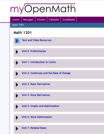Conditional Remix & Share Permitted
CC BY-NC-SA
Rating
0.0 stars

Mathematics 1201, 1206 and 2201 constitute a three-term sequence. Mathematics 1201 is an introduction to calculus: limits and continuity; derivatives and integrals of algebraic, exponential, logarithmic, and trigonometric functions of one variable; methods of numerical approximation, and applications of the derivative to mathematics, physics, engineering, biology, chemistry, and other fields. STEM variant course - Satisfies Pathways Required Core Math and Quantitative Reasoning requirement.

Subject:
Calculus
Mathematics
Material Type:
Activity/Lab
Homework/Assignment
Lecture
Provider:
CUNY
Provider Set:
Brooklyn College
Author:
Amy Wolfe
Brooklyn College Math Faculty
03/08/2021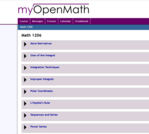Conditional Remix & Share Permitted
CC BY-NC-SA
Rating
0.0 stars

Trigonometric and inverse trigonometric functions, techniques (closed form and numerical) and applications of integration for functions of one variable, improper integrals, l'Hopital's rule, sequences, series, and polar coordinates.

Subject:
Calculus
Mathematics
Material Type:
Activity/Lab
Homework/Assignment
Lecture
Provider:
CUNY
Provider Set:
Brooklyn College
Author:
Amy Wolfe
Brooklyn College Math Faculty
Jeff Suzuki
03/08/2021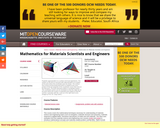Conditional Remix & Share Permitted
CC BY-NC-SA
Rating
0.0 stars

This course covers the mathematical techniques necessary for understanding of materials science and engineering topics such as energetics, materials structure and symmetry, materials response to applied fields, mechanics and physics of solids and soft materials. The class uses examples from the materials science and engineering core courses (3.012 and 3.014) to introduce mathematical concepts and materials-related problem solving skills. Topics include linear algebra and orthonormal basis, eigenvalues and eigenvectors, quadratic forms, tensor operations, symmetry operations, calculus of several variables, introduction to complex analysis, ordinary and partial differential equations, theory of distributions, and fourier analysis. Users may find additional or updated materials at Professor Carter's 3.016 course Web site.

Subject:
Calculus
Mathematics
Material Type:
Full Course
Provider:
M.I.T.
Provider Set:
M.I.T. OpenCourseWare
Author:
Carter, W. Craig
01/01/2005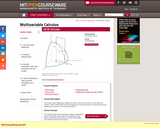Conditional Remix & Share Permitted
CC BY-NC-SA
Rating
0.0 stars

This course covers differential, integral and vector calculus for functions of more than one variable. These mathematical tools and methods are used extensively in the physical sciences, engineering, economics and computer graphics.

Subject:
Calculus
Mathematics
Material Type:
Full Course
Provider:
M.I.T.
Provider Set:
M.I.T. OpenCourseWare
Author:
Auroux, Denis
01/01/2010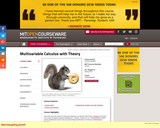Conditional Remix & Share Permitted
CC BY-NC-SA
Rating
0.0 stars

This course is a continuation of 18.014. It covers the same material as 18.02 (Multivariable Calculus), but at a deeper level, emphasizing careful reasoning and understanding of proofs. There is considerable emphasis on linear algebra and vector integral calculus.

Subject:
Calculus
Mathematics
Material Type:
Full Course
Provider:
M.I.T.
Provider Set:
M.I.T. OpenCourseWare
Author:
Breiner, Christine
01/01/2011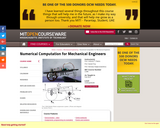Conditional Remix & Share Permitted
CC BY-NC-SA
Rating
0.0 stars

This class introduces elementary programming concepts including variable types, data structures, and flow control. After an introduction to linear algebra and probability, it covers numerical methods relevant to mechanical engineering, including approximation (interpolation, least squares and statistical regression), integration, solution of linear and nonlinear equations, ordinary differential equations, and deterministic and probabilistic approaches. Examples are drawn from mechanical engineering disciplines, in particular from robotics, dynamics, and structural analysis. Assignments require MATLAB programming.

Subject:
Applied Science
Calculus
Engineering
Information Science
Mathematics
Statistics and Probability
Material Type:
Full Course
Provider:
M.I.T.
Provider Set:
M.I.T. OpenCourseWare
Author:
Anthony Patera
Daniel Frey
01/01/2012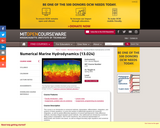Conditional Remix & Share Permitted
CC BY-NC-SA
Rating
0.0 stars

Introduction to numerical methods: interpolation, differentiation, integration, systems of linear equations. Solution of differential equations by numerical integration, partial differential equations of inviscid hydrodynamics: finite difference methods, panel methods. Fast Fourier Transforms. Numerical representation of sea waves. Computation of the motions of ships in waves. Integral boundary layer equations and numerical solutions.

Subject:
Calculus
Mathematics
Material Type:
Full Course
Provider:
M.I.T.
Provider Set:
M.I.T. OpenCourseWare
Author:
Prof. Jerome Milgram
01/01/2003Conditional Remix & Share Permitted
CC BY-NC-SA
Rating
0.0 stars

From the preface, "These are notes for a course in precalculus, as it is taught at New York City College of Technology - CUNY (where it is offered under the course number MAT 1375). Our approach is calculator based. For this, we will use the currently standard TI-84 calculator, and in particular, many of the examples will be explained and solved with it. However, we want to point out that there are also many other calculators that are suitable for the purpose of this course and many of these alternatives have similar functionalities as the calculator that we have chosen to use. An introduction to the TI-84 calculator together with the most common applications needed for this course is provided in appendix A. In the future we may expand on this by providing introductions to other calculators or computer algebra systems."

Subject:
Calculus
Mathematics
Material Type:
Textbook
Provider: